Question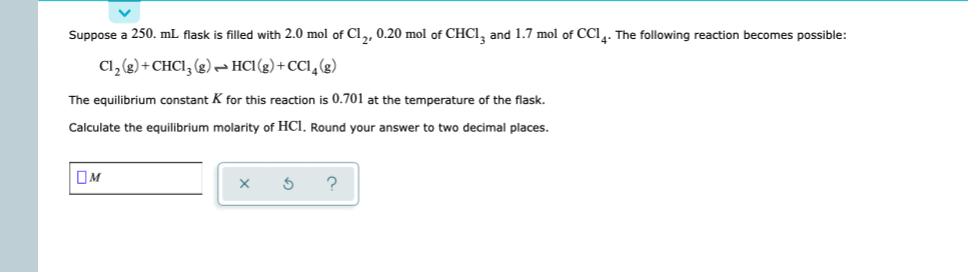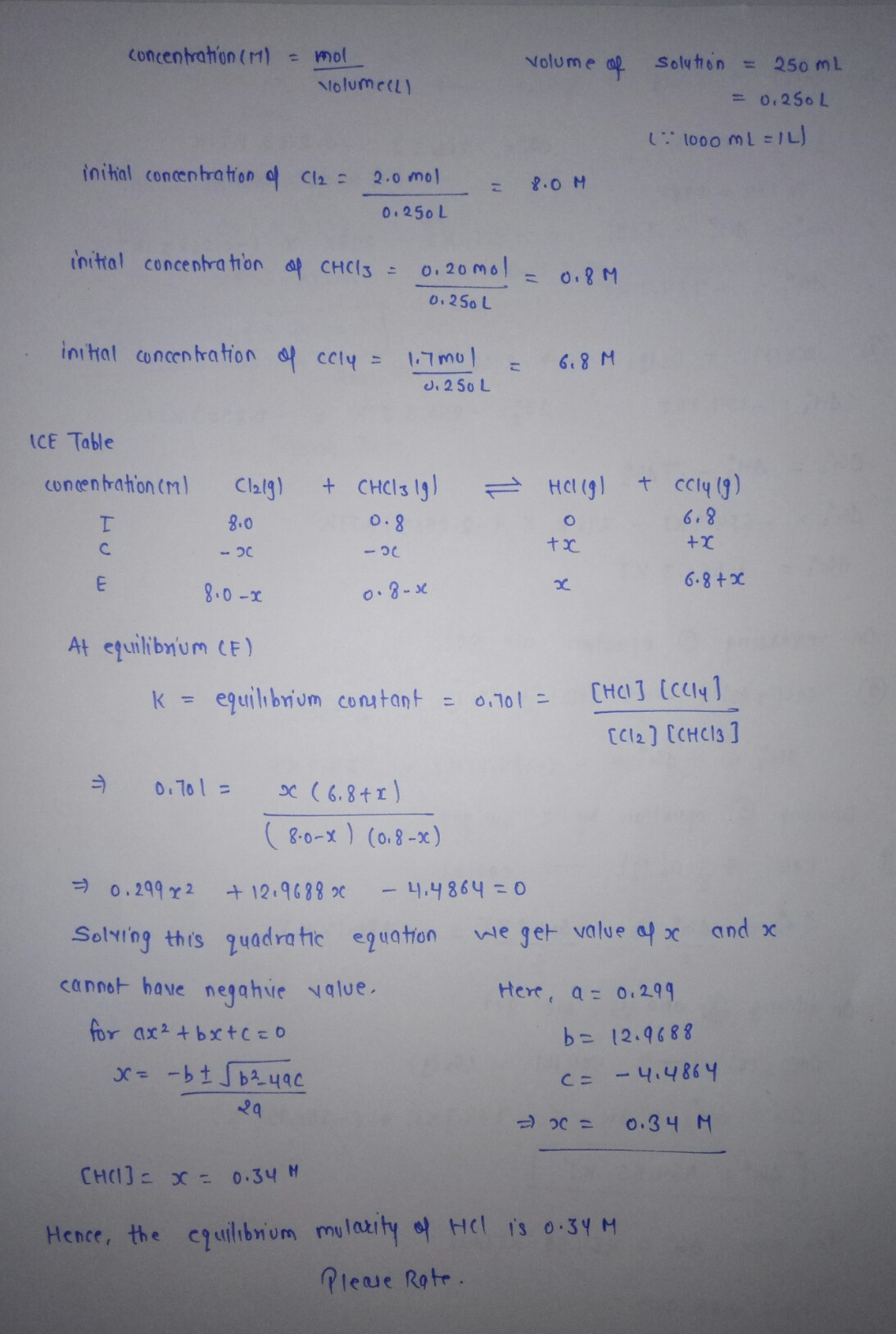#### Earn Coins

Coins can be redeemed for fabulous gifts.

Similar Homework Help Questions
• ### Suppose a 500. mL flask is filled with 1.0 mol of CHCl, 1.6 mol of Hcl...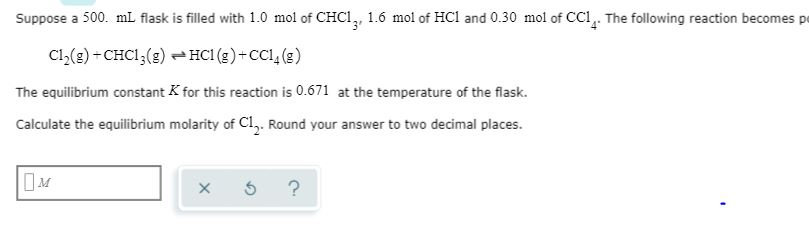Suppose a 500. mL flask is filled with 1.0 mol of CHCl, 1.6 mol of Hcl and 0.30 mol of CCI,. The following reaction becomes p Cl(g)+CHCI (g) HCI (s)+CC () The equilibrium constant K for this reaction is 0.671 at the temperature of the flask. Calculate the equilibrium molarity of Cl,. Round your answer to two decimal places. ?

• ### Suppose a 250. mL flask is filled with 1.2 mol of Cl₂

Suppose a 250. mL flask is filled with 1.2 mol of Cl₂, 1.8 mol of CHCl₃ and 0.90 mol of HCl. The following reaction becomes possible:Cl₂(g)+CHCl₃(g) ⇌ HCl(g)+CCl₄(g)The equilibrium constant K for this reaction is 0.855 at the temperature of the flask.Calculate the equilibrium molarity of HCl. Round your answer to two decimal places.

• ### Suppose a 250. ml flask is filled with 1.5 mol of Cl, and 1.3 mol of...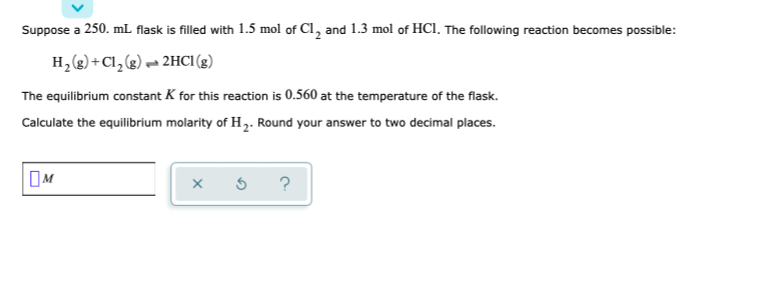Suppose a 250. ml flask is filled with 1.5 mol of Cl, and 1.3 mol of HCl. The following reaction becomes possible: H2(g) + Cl2(g) 2HCl (8) The equilibrium constant K for this reaction is 0.560 at the temperature of the flask. Calculate the equilibrium molarity of H. Round your answer to two decimal places. OM * 5 ?

• ### Suppose a 500 ml flask is filled with 2.0 mol of CHCI, 0.50 mol of HCl...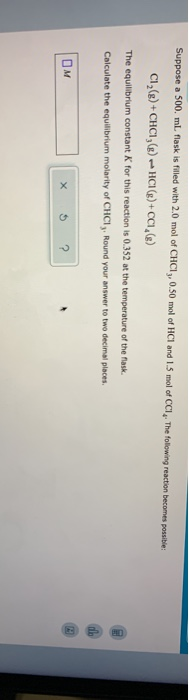Suppose a 500 ml flask is filled with 2.0 mol of CHCI, 0.50 mol of HCl and 1.5 mol of CCI. The following reaction becomes prie CI (8) + CHCI (8) -HCI(g)+CCI (8) The equilibrium constant for this reaction is 0.352 at the temperature of the flask Calculate the equilibrium molarity of CHCI, Round your answer to two decimal places

• ### Suppose a 250. ml flask is filled with 1.6 mol of Cl, and 1.3 mol of...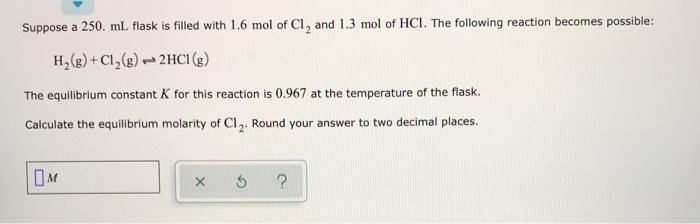Suppose a 250. ml flask is filled with 1.6 mol of Cl, and 1.3 mol of HCI. The following reaction becomes possible: H2(g) + Cl2(g) → 2HCI (g) The equilibrium constant K for this reaction is 0.967 at the temperature of the flask. Calculate the equilibrium molarity of C1. Round your answer to two decimal places. OM I ?

• ### Suppose a 500. ml flask is filled with 1.7 mol of Cl, and 2.0 mol of...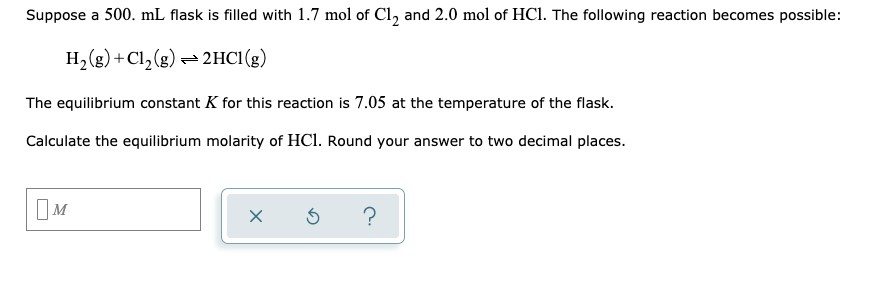Suppose a 500. ml flask is filled with 1.7 mol of Cl, and 2.0 mol of HCl. The following reaction becomes possible: H2(g) +C12(g) + 2HCl (8) The equilibrium constant K for this reaction is 7.05 at the temperature of the flask. Calculate the equilibrium molarity of HCl. Round your answer to two decimal places. x o ?

• ### Suppose a 500. mL flask is filled with 1.9 mol of Cl2, 0.70 mol of HCl...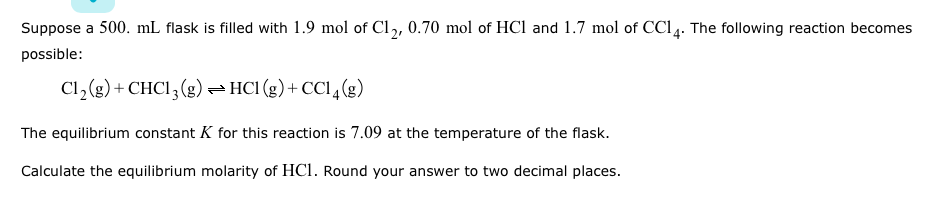Suppose a 500. mL flask is filled with 1.9 mol of Cl2, 0.70 mol of HCl and 1.7 mol of CCI4. The following reaction becomes possible Cl2(g)+ CHCI3)HCI (g)+CCI4g) The equilibrium constant K for this reaction is 7.09 at the temperature of the flask. Calculate the equilibrium molarity of HCl. Round your answer to two decimal places

• ### Suppose a 250. ml flask is filled with 1.7 mol of H, and 0.30 mol of...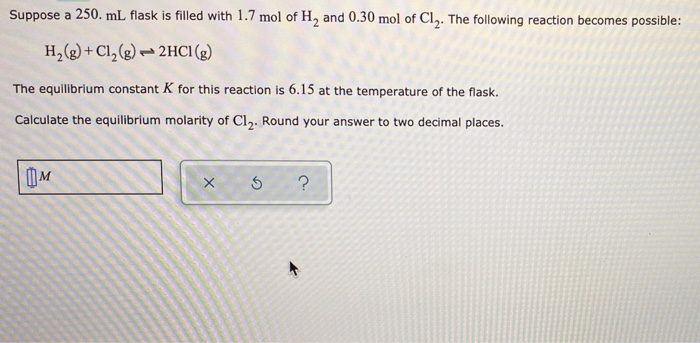Suppose a 250. ml flask is filled with 1.7 mol of H, and 0.30 mol of Cly. The following reaction becomes possible: H2(g) + Cl2(g) - 2HCI(g) The equilibrium constant K for this reaction is 6.15 at the temperature of the flask. Calculate the equilibrium molarity of Cl2. Round your answer to two decimal places. OM xo?

• ### Suppose a 250. ml flask is filled with 0.50 mol of H, and 0.40 mol of...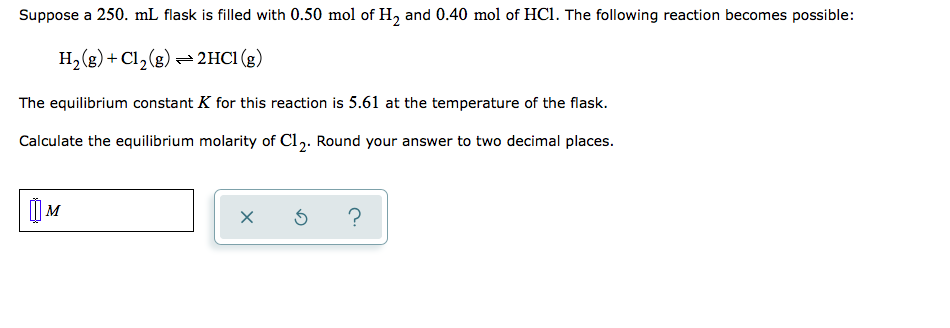Suppose a 250. ml flask is filled with 0.50 mol of H, and 0.40 mol of HCl. The following reaction becomes possible: H2(g) + Cl2(g) = 2HCl (g) The equilibrium constant K for this reaction is 5.61 at the temperature of the flask. Calculate the equilibrium molarity of Cl. Round your answer to two decimal places. TOM xo?

• ### Suppose a 250. mL. flask is filled with 1.8 mol of Cl2 and 0.60 mol of...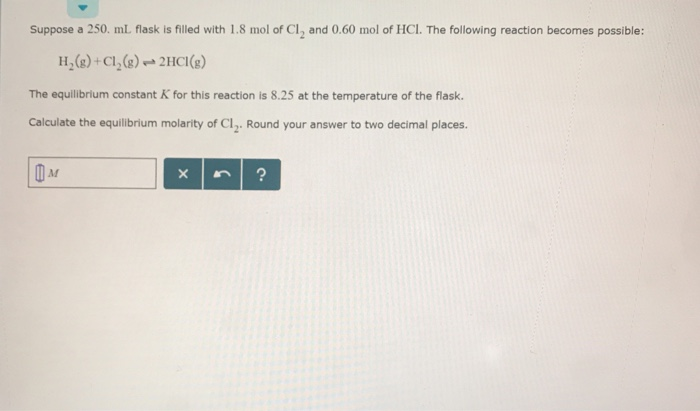Suppose a 250. mL. flask is filled with 1.8 mol of Cl2 and 0.60 mol of HCl. The following reaction becomes possible: H(+Cl()2HCI) The equilibrium constant K for this reaction is 8.25 at the temperature of the flask. Calculate the equilibrium molarity of Cl2. Round your answer to two decimal places.